## RD Sharma Class 9 Solutions Chapter 7 Introduction to Euclid’s Geometry Ex 7.2

These Solutions are part of RD Sharma Class 9 Solutions. Here we have given RD Sharma Class 9 Solutions Chapter 7 Introduction to Euclid’s Geometry Ex 7.2

Other Exercises

Question 1.
Write two solutions for each of the following equations
(i) 3x + 4y = 7
(ii) x = 6y
(iii) x + πy = 4
(iv) $$\frac { 2 }{ 3 }$$ x – y = 4
Solution: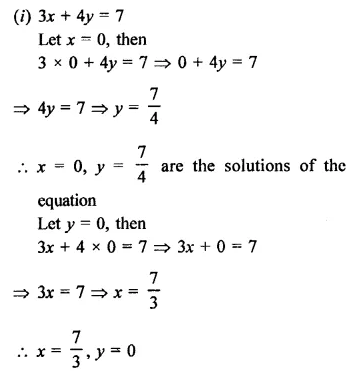(ii)  x = 6y
Let y = 0, then
x = 6 x 0 = 0
∴ x = 0, y = 0
x = 0, y = 0 are the solutions of the equation
Let y= 1, then
x = 6 x 1 = 0                          –
∴ x = 6, y = 1 are the solutions of the equation.
(iii) x + πy = 4
Let x = 4, then
4 + πy = 4
⇒ πy = 4- 4 = 0
∴ y = 0
∴ x = 4, y = 0 are the solutions of the equation
Let x = 0, then
0 + πy = 4 ⇒ πy = 4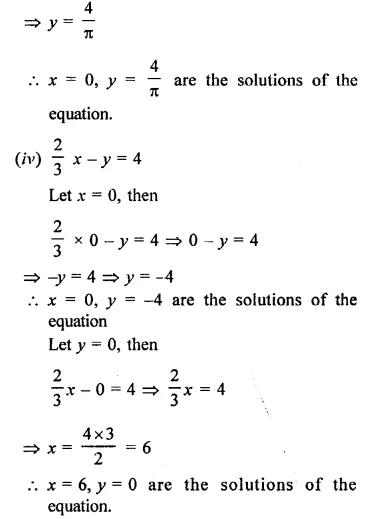Question 2.
Check which of the following are solutions of the equations 2x – y =6 and which are not
(i) (3, 0)
(ii) (0, 6)
(iii) (2,-2)
(iv)($$\sqrt { 3 }$$ ,0)
(v) ($$\frac { 1 }{ 2 }$$ ,-5)
Solution:
Equation is 2x – y = 6
(i) Solution is (3, 0) i.e. x = 3, y = 0
Substituting the value of x and y in the equation
2 x 3 – 0 = 6 ⇒ 6 – 0 = 6
6 = 6
Which is true
∴  (3, 0) is the solutions.
(ii) (0, 6) i.e. x =0, y =6
Substituting the value of x and y in the equation
2 x 0 – 6 = 6 ⇒  0-6 = 6
⇒ -6 = 6  which is not true
∴   (0, 6) is not its solution’
(iii) (2, -2) i.e. x = 2, y = -2
Substituting the value of x and y in the equation
2 x 2 – (-2) = 6 ⇒ 4 + 2 = 6
⇒ 6 = 6 which is true.
∴   (2, -2) is the solution.
(iv) ($$\sqrt { 3 }$$,0) i.e. x = $$\sqrt { 3 }$$ , y = 0,
Substituting the value of x and y in the equations
2 x $$\sqrt { 3 }$$-(0) = 6
⇒ 2$$\sqrt { 3 }$$-0 = 6
⇒  2 $$\sqrt { 3 }$$  6 which is not true
∴ ($$\sqrt { 3 }$$ > 0) is not the solution.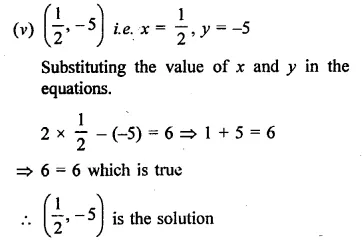Question 3.
If x = -1, y = 2 is a solution of the equation 3x + 4y =k  Find the value of k.
Solution:
x = -1, y = 2
The equation is 3x + 4y = k
Substituting the value of x and y in it
3 x (-1) + 4 (2) = k
⇒ -3+ 8 = k
⇒  5 = k
∴ k = 5

Question 4.
Find the value of λ if x = -λ and y = $$\frac { 5 }{ 2 }$$ is a solution of the equation x + 4y – 7 = 0.
Solution:
x = -λ, y= $$\frac { 5 }{ 2 }$$
Equation is x + 4y – 7 = 0
Substituting the value of x and y,
-λ  + 4 x $$\frac { 5 }{ 2 }$$ -7 = 0
⇒  -λ + 10 – 7 = 0
⇒  -λ +3 = 0
∴  -λ = -3
⇒  λ = 3
Hence λ = 3

Question 5.
If x = 2α + 1 and y = α – 1 is a solution of the equation 2x – 3y + 5 = 0, find the value of α.
Solution:
x = 2α + 1, y = α – 1
are the solution of the equation 2x – 3y + 5 – 0
Substituting the value of x and y
2(2α + 1) -3 (α – 1) + 5 = 0
⇒  4α+ 2-3α+ 3 + 5 = 0
⇒ α+10 = 0
⇒ α = -10
Hence α = -10

Question 6.
If x = 1, and y = 6 is a solution of the equation 8x – ay + a2 = 0, find the values of a.
Solution:
x = 1, y = 6 is a solution of the equation
8x – ay + a2 = 0
Substituting the value of x and y,
8 x 1-a x 6 + a2 = o
⇒  8 – 6a + a2 = 0
⇒  a2 – 6a + 8 = 0
⇒  a2 – 2a -4a + 8 = 0
⇒  a (a – 2) – 4 (a – 2) = 0
⇒  (a – 2) (a – 4) = 0
Either a – 2 = 0, then a = 2
or a – 4 = 0, then a = 4
Hence a = 2, 4

Question 7.
Write two solutions of the form x = 0, y = a and x = b, y = 0 for each of the following equations.
(i) 5x – 2y = 10
(ii) -4x + 3y = 12
(iii) 2x + 3y = 24
Solution:
(i)  5x – 2y = 10
Let x = 0, then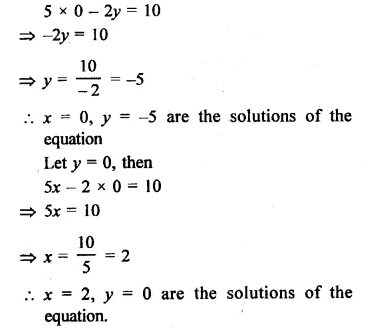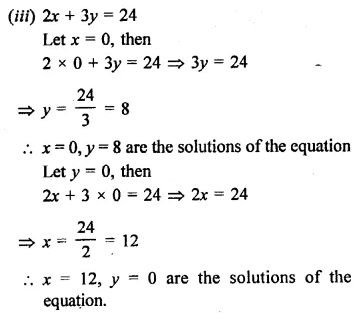Hope given RD Sharma Class 9 Solutions Chapter 7 Introduction to Euclid’s Geometry Ex 7.2 are helpful to complete your math homework.

If you have any doubts, please comment below. Learn Insta try to provide online math tutoring for you.